Integro-differential equation

An equation containing the unknown function under the sign of both differential and integral operations. Integral equations and differential equations are also integro-differential equations.

Linear integro-differential equations.

Letbe a given function of one variable, let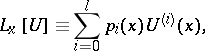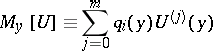be differential expressions with sufficiently smooth coefficients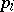and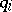on, and letbe a known function that is sufficiently smooth on the square. An equation of the form(1)

is called a linear integro-differential equation;is a parameter. If in (1) the function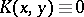for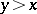, then (1) is called an integro-differential equation with variable integration limits; it can be written in the form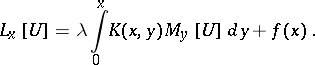(2)

For (1) and (2) one may pose the Cauchy problem (find the solution satisfying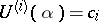,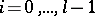, where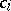are given numbers,is the order of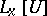, and), as well as various boundary value problems (e.g., the problem of periodic solutions). In a number of cases (cf. , ), problems for (1) and (2) can be simplified, or even reduced, to, respectively, Fredholm integral equations of the second kind or Volterra equations (cf. also Fredholm equation; Volterra equation). At the same time, a number of specific phenomena arise for integro-differential equations that are not characteristic for differential or integral equations.

The simplest non-linear integro-differential equation has the formThe contracting-mapping principle, the Schauder method, as well as other methods of non-linear functional analysis, are applied in investigations of this equation.

Questions of stability of solutions, eigen-function expansions, asymptotic expansions in a small parameter, etc., can be studied for integro-differential equations. Partial integro-differential and integro-differential equations with multiple integrals are often encountered in practice. The Boltzmann and Kolmogorov–Feller equations are examples of these.